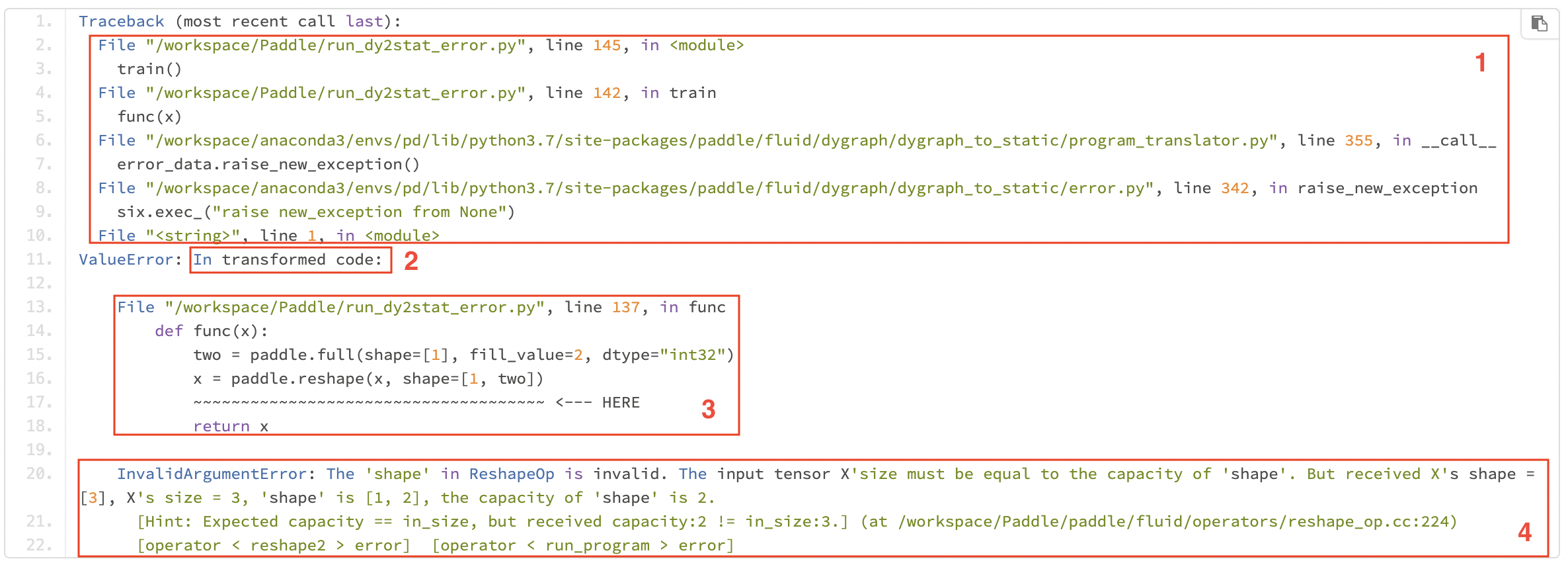# 报错调试¶

## 一、动转静报错日志¶

### 1.1 错误日志怎么看¶

```import paddle
import numpy as np

def func(x):
return x

def train():
func(x)

if __name__ == '__main__':
train()
```• 原生的 Python 报错栈：如 1 中的前两行所示，表示`/workspace/Paddle/run_dy2stat_error.py`文件第 145 行调用的函数`train()`导致的后续一系列报错。

• 动转静报错栈起始标志位`In transformed code`，表示动转静报错信息栈，指运行转换后的代码时的报错信息。实际场景中，可以直接搜索`In transformed code`关键字，从这一行以下开始看报错日志即可。

• 用户代码报错栈：隐藏了框架层面的无用的报错信息，突用户代码报错栈。我们在出错代码下添加了波浪线和 HERE 指示词来提示具体的出错位置，并扩展了出错行代码上下文，帮助你快速定位出错位置。如上图 3 中所示，可以看出最后出错的用户代码为`x = paddle.reshape(x, shape=[1, two])`

• 框架层面报错信息：提供了静态图组网报错信息。一般可以直接根据最后三行的信息，定位具体是在生成哪个 OpDesc 时报的错误，一般是与执行此 Op 的 infershape 逻辑报的错误。 如上报错信息表明是 reshape Op 出错，出错原因是 tensor x 的 shape 为，将其 reshape 为[1, 2]是不被允许的。

NOTE：在某些场景下，会识别报错类型并给出修改建议，如下图所示。`Revise suggestion`下面是出错的排查建议，你可以根据建议对代码进行排查修改。### 1.2 报错信息定制化展示¶

#### 1.2.1 未经动转静报错模块处理的原生报错信息¶

```import os
os.environ["TRANSLATOR_DISABLE_NEW_ERROR"] = '1'
```#### 1.2.2 C++报错栈¶## 二、调试方法¶

### 2.1 pdb 调试¶

pdb 是 Python 中的一个模块，该模块定义了一个交互式 Python 源代码调试器。它支持在源码行间设置断点和单步执行，列出源代码和变量，运行 Python 代码等。

#### 2.1.1 调试步骤¶

• step1：在想要进行调试的代码前插入`import pdb; pdb.set_trace()`开启 pdb 调试。

```import paddle
import numpy as np

def func(x):
import pdb; pdb.set_trace()       # <------ 开启 pdb 调试
return x

func(np.ones().astype("int32"))
```
• step2：正常运行.py 文件，在终端会出现下面类似结果，在`(Pdb)`位置后输入相应的 pdb 命令进行调试。

```> /tmp/tmpm0iw5b5d.py(9)func()
-> two = paddle.full(shape=, fill_value=2, dtype='int32')
(Pdb)
```
• step3：在 pdb 交互模式下输入 l、p 等命令可以查看动转静后静态图相应的代码、变量，进而排查相关的问题。

```> /tmp/tmpm0iw5b5d.py(9)func()
-> two = paddle.full(shape=, fill_value=2, dtype='int32')
(Pdb) l
4     import numpy as np
5     def func(x):
7         import pdb
8         pdb.set_trace()
9  ->     two = paddle.full(shape=, fill_value=2, dtype='int32')
10         x = paddle.reshape(x, shape=[1, two])
11         return x
[EOF]
(Pdb) p x
(Pdb)
```

#### 2.1.2 常用命令¶### 2.2 打印转换后的静态图代码¶

#### 2.2.1 set_code_level() 或 TRANSLATOR_CODE_LEVEL¶

```import paddle
import numpy as np

def func(x):
if x > 3:
x = x - 1
return x

paddle.jit.set_code_level() # 也可设置 os.environ["TRANSLATOR_CODE_LEVEL"] = '100'，效果相同
func(np.ones())
```

#### 2.2.2 被装饰后的函数的 code 属性¶

```import paddle
import numpy as np

def func(x):
if x > 3:
x = x - 1
return x

func(np.ones())
print(func.code)
```

```def func(x):

def true_fn_0(x):
x = x - 1
return x

def false_fn_0(x):
return x
x = paddle.jit.dy2static.convert_ifelse(x > 3, true_fn_0, false_fn_0, (
x,), (x,), (x,))
return x
```

### 2.3 使用 print 查看变量¶

```import paddle
import numpy as np

def func(x):

print(x)
print("Here call print function.")

if len(x) > 3:
x = x - 1
else:
return x

func(np.ones())
```

```Variable: assign_0.tmp_0
- lod: {}
- place: CUDAPlace(0)
- shape: 
- layout: NCHW
- dtype: double
- data: 
```

### 2.4 日志打印¶

• 0: 无日志

• 1: 包括了动转静转化流程的信息，如转换前的源码、转换的可调用对象

• 2: 包括以上信息，还包括更详细函数转化日志

• 3: 包括以上信息，以及更详细的动转静日志

```import paddle
import numpy as np
import os

def func(x):
if len(x) > 3:
x = x - 1
else:
return x

# 或者设置 os.environ["TRANSLATOR_VERBOSITY"] = '3'
func(np.ones())
```

```Sun Sep 26 08:50:20 Dynamic-to-Static INFO: (Level 1) Source code:
def func(x):
if len(x) > 3:
x = x - 1
else:
return x

Sun Sep 26 08:50:20 Dynamic-to-Static INFO: (Level 1) Convert callable object: convert <built-in function len>.
```

## 三、快速确定问题原因¶

### 3.1 (NotFound) Input("X")¶

```RuntimeError: (NotFound) Input("Filter") of ConvOp should not be null.
[Hint: Expected ctx->HasInputs("Filter") == true, but received ctx->HasInputs("Filter"):0 != true:1.]
[operator < conv2d > error]
```

• 首先确认代码所在的 sublayer 是否继承了 nn.Layer

• 此行代码所在函数是否绕开了 forward 函数，单独调用的（2.1 版本之前）

• 查看是 Tensor 还是 Variable 类型，可以通过 pdb 交互式调试

### 3.2 Expected input_dims[i] == input_dims¶

```[Hint: Expected input_dims[i] == input_dims, but received input_dims[i]:-1, -1 != input_dims:16, -1.]
[operator < xxx_op > error]
```

• 代码层面，判断是否是上游使用了 reshape 导致 -1 的污染性传播

• 可以结合调试技巧，判断是否是某个 API 的输出 shape 在动静态图下有 diff 行为

### 3.3 desc->CheckGuards() == true¶

```[Hint: Expected desc->CheckGuards() == true, but received desc->CheckGuards():0 != true: 1.]
```• 模型代码是否存在上述复杂的 Tensor slice 切片操作

• 推荐使用 paddle.slice 接口替换复杂的 Tensor slice 操作

### 3.4 Segment Fault¶

• 每个 sublayer 是否继承了 nn.Layer

### 3.5 Container 的使用建议¶

• ParameterList

```class MyLayer(paddle.nn.Layer):
def __init__(self, num_stacked_param):
super().__init__()

# 此用法下，MyLayer.parameters() 返回为空
self.params = [w1, w2]                            # <----- 错误用法

self.params = paddle.nn.ParameterList([w1, w2])   # <----- 正确用法
```
• LayerList

```class MyLayer(paddle.nn.Layer):
def __init__(self):
super().__init__()